# Problem 55500. Easy Sequences 71: A Nonlinear Function with Quadratic Domain

Consider the function: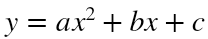The outputs of this function are fed as domain to another function:where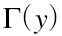, is the Gamma Function.
Given the values of a, b, c, and a limit n, find the all values of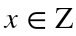, so that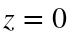and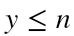. For example if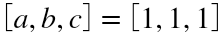, and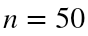, the only integer values of x that will satisfy the above criteria are: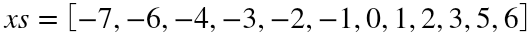.
Please present the final output as a unique row vector sorted ascending.
-----------------
NOTE: Some MATLAB's built-in math functions may only give approximate values.

### Solution Stats

100.0% Correct | 0.0% Incorrect
Last Solution submitted on Sep 11, 2022

### Community Treasure Hunt

Find the treasures in MATLAB Central and discover how the community can help you!

Start Hunting!